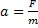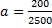# Laws of Motion topic -- Car being decelerated by a headwind

Barclay

## Homework Statement

A car with mass 2500 kilograms travels along a straight level road at 90 km/h. A headwind begins to blow with a force of 200 N causing the car to slow down. Calculate the car’s deceleration.

## Homework Equations

The book uses the formula F =ma so3. Book's solution= 0.08 m/s2

My confusion with the calculation comes from not knowing why the speed of the car is not considered. It’s a 2500 kg car driving at 90km/h so must have a force or a momentum that is important. Why is the car's speed not considered?

The second part of the question says

The car travelling at a steady speed collides with a stationary traffic cone of mass 10 kg. The traffic on accelerates in the direction of the car’s motion at 29 m/s2.
Calculate the force applied to the traffic cone by the car.

Book says: Calculation is F =ma = 10 x 29 = 290N

Book asks: What is the force applied by the cone to the car in the collision (hint in the paper is: Newton’s third law of motion).

Answer in the book is 290N. But shouldn’t the answer be -290N because “to every action is an equal and opposite reaction”.

•haruspex

Homework Helper
Re deceleration: it is meant to be a simple exercise. No mention of cruise control either :)
so apply Newton 1
Re cone: Acceleration is in the direction of the car's motion, which (apparently) is chosen to be the positive direction (it says 29 m/s2, not -29 m/s2). Force applied by the car to the cone is then also considered positive. Force of cone on car is then -290 N.

Joshua L
For the first part of the question, the speed of the car that the question gives you is a red-herring; it tries to tricks students into believing that knowing the initial speed is important in this situation. Since you already have the the net force and the mass of the car, you can easily solve for the deceleration.

Barclay
Force of cone on car is then -290 N.

So I'm correct ??